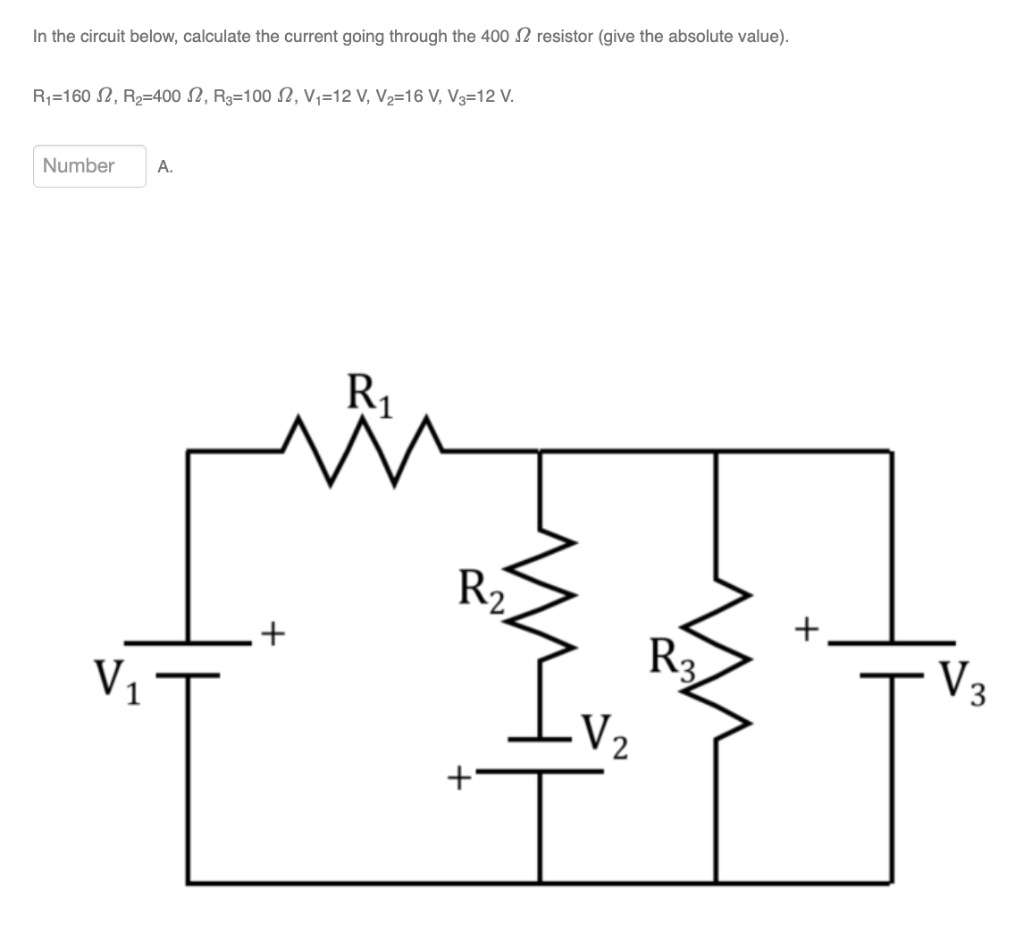# Question In the circuit below, calculate the current going through the 400 12 resistor (give the absolute value). R1=160 2, R2=400 12, Rg=1002, Vi=12 V, V2=16 V, V3=12 V. Number A. R1 M R2 + + R3 V mit V + 1